Thermodynamics and Propulsion

# 18.2 Heat Transfer From a Fin

Fins are used in a large number of applications to increase the heat transfer from surfaces. Typically, the fin material has a high thermal conductivity. The fin is exposed to a flowing fluid, which cools or heats it, with the high thermal conductivity allowing increased heat being conducted from the wall through the fin. The design of cooling fins is encountered in many situations and we thus examine heat transfer in a fin as a way of defining some criteria for design.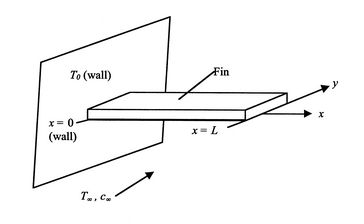A model configuration is shown in Figure 18.3. The fin is of length. The other parameters of the problem are indicated. The fluid has velocity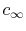and temperature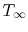. We assume (using the Reynolds analogy or other approach) that the heat transfer coefficient for the fin is known and has the value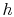. The end of the fin can have a different heat transfer coefficient, which we can call.

The approach taken will be quasi-one-dimensional, in that the temperature in the fin will be assumed to be a function of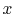only. This may seem a drastic simplification, and it needs some explanation. With a fin cross-section equal to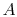and a perimeter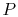, the characteristic dimension in the transverse direction is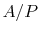(For a circular fin, for example,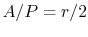). The regime of interest will be taken to be that for which the Biot number is much less than unity,, which is a realistic approximation in practice.

The physical content of this approximation can be seen from the following. Heat transfer per unit area out of the fin to the fluid is roughly of magnitudeper unit area. The heat transfer per unit area within the fin in the transverse direction is (again in the same approximate terms)where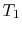is an internal temperature. These two quantities must be of the same magnitude. If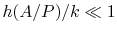, then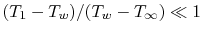. In other words, if, there is a much larger capability for heat transfer per unit area across the fin than there is between the fin and the fluid, and thus little variation in temperature inside the fin in the transverse direction. To emphasize the point, consider the limiting case of zero heat transfer to the fluid, i.e., an insulated fin. Under these conditions, the temperature within the fin would be uniform and equal to the wall temperature.If there is little variation in temperature across the fin, an appropriate model is to say that the temperature within the fin is a function ofonly,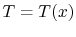, and use a quasi-one-dimensional approach. To do this, consider an element,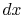, of the fin as shown in Figure 18.4. There is heat flow of magnitude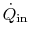at the left-hand side and heat flow out of magnitude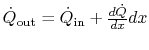at the right hand side. There is also heat transfer around the perimeter on the top, bottom, and sides of the fin. From a quasi-one-dimensional point of view, this is a situation similar to that with internal heat sources, but here, for a cooling fin, in each elemental slice of thicknessthere is essentially a heat sink of magnitude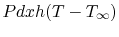, where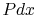is the area for heat transfer to the fluid.

The heat balance for the element in Figure 18.4 can be written in terms of the heat flux using, for a fin of constant area: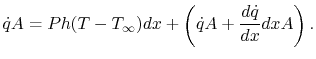(18..7)

From Equation (18.7) we obtainIn terms of the temperature distribution,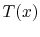: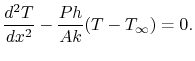(18..8)

The quantity of interest is the temperature difference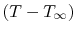, and we can change variables to put Equation (18.8) in terms of this quantity using the substitution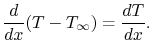Equation (18.8) can therefore be written as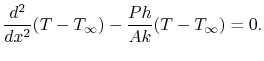(18..9)

Equation (18.9) describes the temperature variation along the fin. It is a second order equation and needs two boundary conditions. The first of these is that the temperature at the end of the fin that joins the wall is equal to the wall temperature. (Does this sound plausible? Why or why not?)The second boundary condition is at the other end of the fin. We will assume that the heat transfer from this end is negligible18.1. The boundary condition atisThe last step is to work in terms of non-dimensional variables to obtain a more compact description. In this we defineas, where the values ofrange from zero to one. We also define, where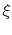also ranges over zero to one. The relation between derivatives that is needed to cast the equation in terms ofis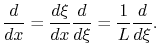Equation (18.9) can be written in this dimensionless form as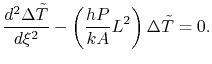(18..10)

There is one non-dimensional parameter in Equation (18.10), which we will call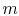and define byThe equation for the temperature distribution we have obtained isThis second order equation has the solution(Try it and see). The boundary condition at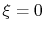isThe boundary condition at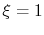is that the temperature gradient is zero orSolving the two equations given by the boundary conditions forandgives an expression forin terms of the hyperbolic cosine or: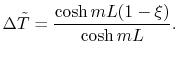(18..11)

This is the solution to Equation (18.10) for a fin with no heat transfer at the tip. In terms of the actual heat transfer parameters it is written as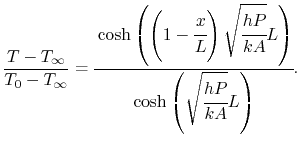(18..12)

The amount of heat removed from the wall due to the fin, which is the quantity of interest, can be found by differentiating the temperature and evaluating the derivative at the wall,:or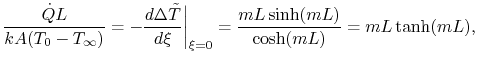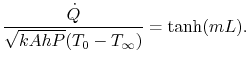(18..13)

The solution is plotted in Figure 18.5, which is taken from the book by Lienhard. Several features of the solution should be noted. First, one does not need fins which have a length such thatis much greater than 3. Second, the assumption about no heat transfer at the end begins to be inappropriate asgets smaller than 3, so for very short fins the simple expression above would not be a good estimate. We will see below how large the error is.Muddy Points

Why did you change the variable and write the derivative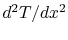as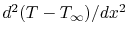in the equation for heat transfer in the fin? (MP 18.2)

What types of devices use heat transfer fins? (MP 18.3)

Why did the Stegosaurus have cooling fins? Could the stegosaurus have heating fins?'' (MP 18.4)

UnifiedTP